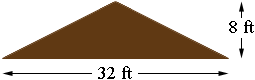SEARCH HOMEMath Central Quandaries & QueriesQuestion from Roy: I need to know how many cubic yards are in a berm 100 ft long 8 ft high and 32 ft wide at the bottom? Looking from the end of the berm it would look like a triangle. Thanks for the help.Hi Roy,

I am going to assume the top of the berm is centered above the center of the 32 foot base, as in the diagram.The volume of the dirt in the berm is the area of the triangular cross section, times the length. The area of a triangle is one half of the length of the base, times the height which in your case is $\frac12 \times 32 \times 8 = 128$ square feet. Thus the volume of the berm is $64 \times 100 = 6400$ cubic feet. One yard is 3 feet so a cubic yard is 3 by 3 by 3 or $3 \times 3 \times 3 = 27$ cubic feet. Thus the volume of your berm is $\frac{6400}{27} = 237$ cubic yards.

PennyMath Central is supported by the University of Regina and The Pacific Institute for the Mathematical Sciences.